## MethodsS2C Home « Methods

We have used methods throughout the lessons so far, so its time for a thorough investigation of what we can do with these members. There are two types of methods available in Java static and non-static methods. The general mechanics are the same but static methods apply to the class as a whole, whereas non-static methods generally apply to an instance of the class. We will discuss the vagaries of static methods in the Static Members lesson later in the section. This lesson is about using methods and for that we will be using a combination of both within our code examples. Lets start by looking at the components of a method: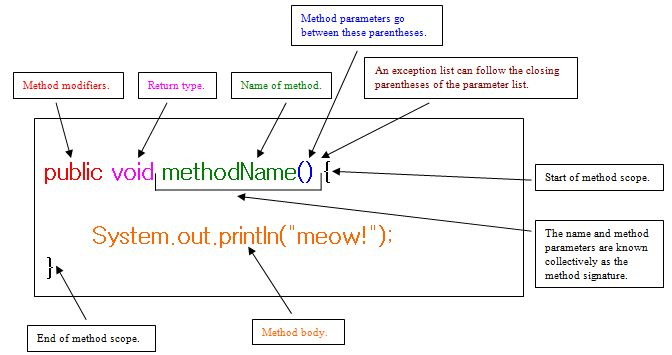Method declarations can have up to six components but the only required elements are the method's return type, the method name, a pair of parenthesis `()` and a body between braces `{}`. Following is the order the components must appear in when used.

1. Modifiers — such as `public` as used here are discussed in the Encapsulation lesson along with the other access modifiers and `static` which we will discuss later in this section in the Static Members lesson. There are also other modifiers which we will learn about later.
2. The return type — which is the data type of the value returned by the method, or `void` if the method doesn't return any value.
3. The method name — which must be a valid identifier as discussed in the Variables in Java section of the Primitive Variables lesson. By convention, method names should be a verb in lowercase or multiple words that begin with a verb in lowercase, followed by adjectives, nouns, etc. in camel case.
4. The parameter list - A comma-delimited list of input parameters, preceded by their data types, enclosed by parentheses. If there are no parameters, you must use empty parentheses. The arguments passed when we call a method are received into the parameter list are within scope as discussed in the Defining A Scope section of the Method Scope lesson.
5. An exception list — Which will be discussed when we get to look at exceptions.
6. The method body - The method's code, statements, local variable declaration etc, goes in the body which is enclosed between braces.

### Passing Arguments To A Method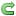Top

Before we look at this lets clear up some terminology about arguments and parameters. Arguments are what we pass to a method whereas parameters are what we receive the arguments into. We have already seen with our use of the `main()` method how to pass an argument to a method and we also coded our own `printBits` method in the Bitwise Operators lesson. Both these methods accept a single argument into their parameter list, but Java has no restriction on the amount of parameters we can code into a method. Lets code a `Tiger` class with a new method that accepts two parameters:

``````
/*
A Tiger Class
*/
public class Tiger {
String name, colour;
int age;

void tigerMannerisms(String action, int times) {  // Our new method with 2 parameters
System.out.println("Our tiger " + action + " " + times + " times a day.");
}
}

``````

Save and compile the file in directory   c:\_ObjectsAndClasses in the usual way. Lets write a new test class for our `Tiger` class.

``````
/*
Test Class for Tiger
*/
public class TigerTest {

public static void main (String[] args) {
Tiger tony = new Tiger();  // Create a Tiger instance
tony.tigerMannerisms("eats food", 4);  // Pass values
String tiggerAction = "runs up trees";
int tiggerTimes = 2;
tony.tigerMannerisms(tiggerAction , tiggerTimes );  // Pass types
tony.tigerMannerisms(tony.name, tony.age );  // Pass instance variables
}
}

``````

Save, compile and run the file in directory   c:\_ObjectsAndClasses in the usual way.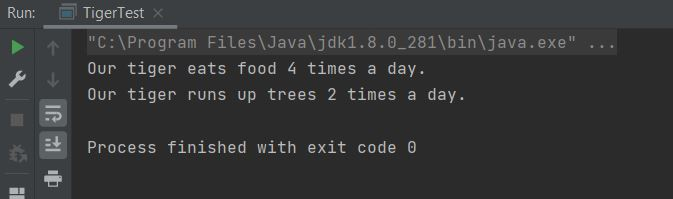The above screenshot shows the output of running our `Tiger` class. We created a `Tiger` instance and passed two arguments to the `tigerMannerisms` method in various ways.

### Returning Values From A MethodTop

Up until now all our methods have been declared using the `void` return type which denotes that the method doesn't return any value. We can declare methods with a return type and if we do we must return a value compatible with that type or covariant thereof, using the `return` keyword. Lets see this in action :

``````
/*
Test class for maths stuff
*/
public class MathsStuff {

public static void main (String[] args) {
int aSquare = squareNumber(5);   // The return value will go into aSquare
System.out.println(aSquare);
}

/*
A method that squares and returns the passed integer
*/
static int squareNumber(int number) {
int square = number * number;
return square;  // Here we use the return keyword to pass back a value
}
}

``````

Save, compile and run the file in directory   c:\_ObjectsAndClasses in the usual way.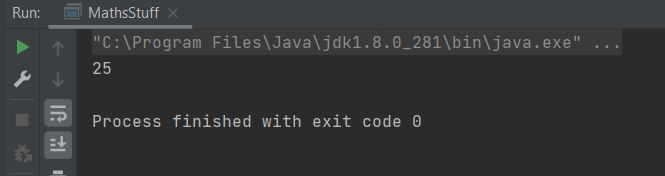The above screenshot shows the output of running our `MathsStuff` class. The return value from the `squareNumber()` method gets assigned to the `aSquare` primitive.

### Final ParametersTop

There might be a come a time when we want to pass a variable to a method that we want to remain constant during the scope of the method. When we write our parameter lists we can prefix our variables with the `final` keyword. This makes the passed value a constant in the method, which cannot be changed, in fact trying to do so will cause a compiler error. The following code illustrates this:

``````
/*
A Class
*/
public class A {

final void usingFinalParam (final int i) {
i = 0;
}
}

``````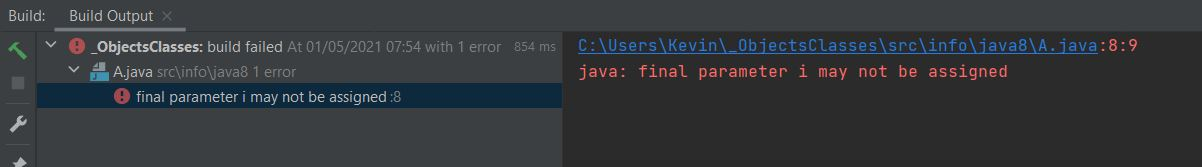The above screenshot shows the result of trying to compile class `A`.

You can also use the `final` keyword to create final local variables, final instance variables, java constants and for preventing inheritance/overriding.

### Overloaded MethodsTop

You can also use the same method name more than once and this is known as overloading. When using overloading each method must have different parameter lists so the compiler knows which method to call. Having the same parameter types is fine as long as the order differs. Lets see this in action :

``````
/*
Test class for maths stuff
*/
public class MathsStuff {

public static void main (String[] args) {
int aSquare = squareNumber(5);   // The return value will go into aSquare
System.out.println(aSquare);
float aFracSquare = squareNumber(12.2F);   // The return value will go into aFracSquare
System.out.println(aFracSquare);
}

/*
A method that squares and returns the passed integer
*/
static int squareNumber(int number) {
int square = number * number;
return square;  // Here we use the return keyword to pass back a value
}

/*
A method that squares and returns the passed float
*/
static float squareNumber(float number) {
float square = number * number;
return square;  // Here we use the return keyword to pass back a value
}
}

``````

Save, compile and run the file in directory   c:\_ObjectsAndClasses in the usual way.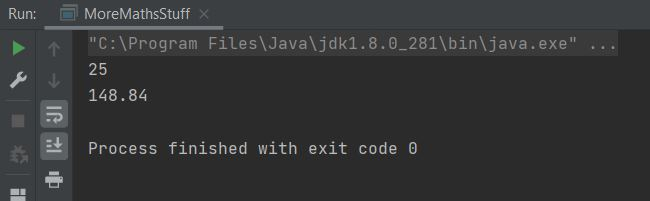The above screenshot shows the output of running our `MathsStuff` class. The compiler knows which method to call from the argument list.

### Pass-By-ValueTop

When we pass an argument to a method we are actually passing a copy of that argument and this is known as pass-by-value. To explore what this means lets look at some code:

``````
/*
A Dog Class
*/
public class Dog {
String name, colour;

void dogLooks(String name, String colour) {
System.out.println("Our dog " + name + " is " + colour + "(input arguments)");
name = "zzz";
colour = "zzz";
System.out.println("Our dog " + name + " is " + colour + "(changed arguments)");
}
}

``````

Save and compile the file in directory   c:\_ObjectsAndClasses in the usual way. Lets write a test class for our `Dog` class.

``````
/*
Test Class for Dog
*/
public class TestDog {

public static void main (String[] args) {
Dog doggy = new Dog();  // Create a Dog instance
String name = "rover";
String colour = "black";
doggy.dogLooks(name, colour);
System.out.println("Our dog " + name + " is " + colour + "(after call to dogLooks() )");
}
}

``````

Save, compile and run the file in directory   c:\_ObjectsAndClasses in the usual way.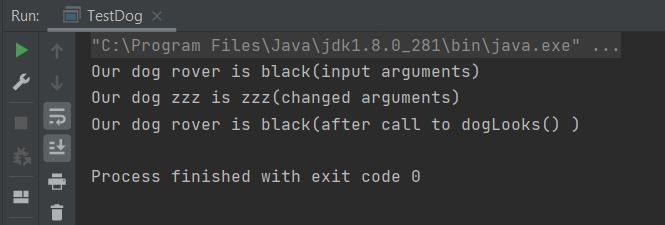The above screenshot shows the output of running our `TestDog` class. As you can see even though we change the parameters in the `dogLooks` method, when we return and print the passed arguments they are the same as before the call to `dogLooks`. This is because Java made a copy of the variables and passed them into our method. Therefore any changes to the variables were made to the copies and not to the originating argument list.

#### Pass-By-Value Using Reference Variables

So what if we pass a reference variable to a method? If you recall our objects live on The Heap and we access them through their reference variable. Lets look into this:

``````
/*
Another Dog Class
*/
public class Dog2 {
String name, colour;

void dogLooks(Dog2 dog) {
System.out.println("Our dog " + dog.name + " is " + dog.colour + "(input arguments)");
dog.name = "zzz";
dog.colour = "zzz";
System.out.println("Our dog " + dog.name + " is " + dog.colour + "(changed arguments)");
}
}

``````

Save and compile the file in directory   c:\_ObjectsAndClasses in the usual way. Lets write a test class for our `Dog2` class.

``````
/*
Test Class for Dog2
*/
public class TestDog2 {

public static void main (String[] args) {
Dog2 doggy = new Dog2();  // Create a Dog instance
doggy.name = "rover";
doggy.colour = "black";
doggy.dogLooks(doggy);  // Pass a reference variable to a method
System.out.println("Our dog " + doggy.name + " is " + doggy.colour
+ "(after call to dogLooks() )");
}
}

``````

Save, compile and run the file in directory   c:\_ObjectsAndClasses in the usual way.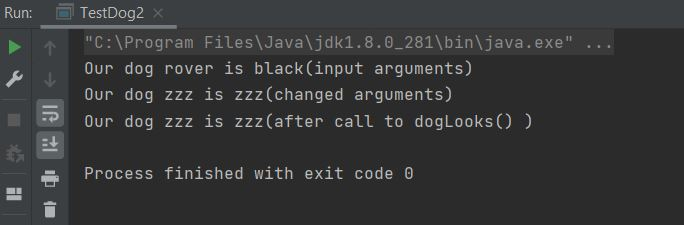The above screenshot shows the output of running our `TestDog2` class. As you can see when we change the instance variables in the method the values have changed when we return. It is still passing a copy of the reference variable to the method, but we are updating the actual instance variables on The Heap. So the changes in the method persist when the `dogLooks` method ends.

### Variable-Length ArgumentsTop

Variable-length arguments (varargs) were introduced in Java6 to give developers a way of defining a method with a parameter list that allowed a variable amount of arguments to be passed to it. In all previous versions of Java the only way to do this was through overloading or using an array. Overloading works fine when you have a small amount of argument combinations to pass and thus a small amount of overloaded methods. Using an array isn't the most elegant solution to this problem either as we have to declare an array size and we have no way of knowing the amount of arguments each time the method is called. So Java6 came with varargs which allows us to define a parameter list that can vary dependant upon the arguments passed to it. We specify a varargs parameter using an ellipsis `...` between the type and name. Following are a few examples of the syntax:

``````
int ... a
boolean ... b
String ... c

``````

The syntax tells the compiler that the method can be called with `0` or more arguments. The compiler also implicitly declares the defined varargs as an array of the correct size for the arguments and as such we can use array syntax on the parameter within the method.

There are a couple of rules about using varargs in our code:

1. When using varargs in a parameter list, the varargs parameter must be the last entry in the parameter list.
2. You can only have one varargs parameter in a parameter list.

Lets look at an example of using varargs:

``````
/*
Test class for maths stuff
*/
public class NumberAdder {

public static void main (String[] args) {
int a = 12;
int b = 100;
int c = 97;
addNumbers();  // Call with no arguments
addNumbers(a);  // Call with 1 arguments
addNumbers(a, b);  // Call with 2 arguments
addNumbers(a, b, c);  // Call with 3 arguments
}

/*
A method that adds up the integers in a varargs list
*/
static void addNumbers(int ... number) {
int adder = 0;
for (int i=0; i<number.length; i++) {
System.out.println("Array position: " + i + ": " + number[i]);
}
System.out.println("Total = " + adder + "\n");
}
}

``````

Save, compile and run the file in directory   c:\_ObjectsAndClasses in the usual way.The above screenshot shows the output of running our `NumberAdder` class. As you can see we can pass a variable number of arguments to the method.

We can overload methods that use varargs just like any other method:

``````
/*
Test class for maths stuff
*/
public class NumberAdder2 {

public static void main (String[] args) {
int a = 12;
addNumbers();  // Call with no arguments
addNumbers(a);  // Call with 1 arguments
float d = 12.1F;
addNumbers();  // Call with no arguments
addNumbers(d);  // Call with 1 arguments
}

/*
A method that adds up the integers in a varargs list
*/
static void addNumbers(int ... number) {
int adder = 0;
for (int i=0; i<number.length; i++) {
System.out.println("Array position: " + i + ": " + number[i]);
}
System.out.println("Total = " + adder + "\n");
}

/*
A method that adds up the floats in a varargs list
*/
static void addNumbers(float ... number) {
int adder = 0;
for (int i=0; i<number.length; i++) {
System.out.println("Array position: " + i + ": " + number[i]);
}
System.out.println("Total = " + adder + "\n");
}
}

``````

Save, compile and run the file in directory   c:\_ObjectsAndClasses in the usual way.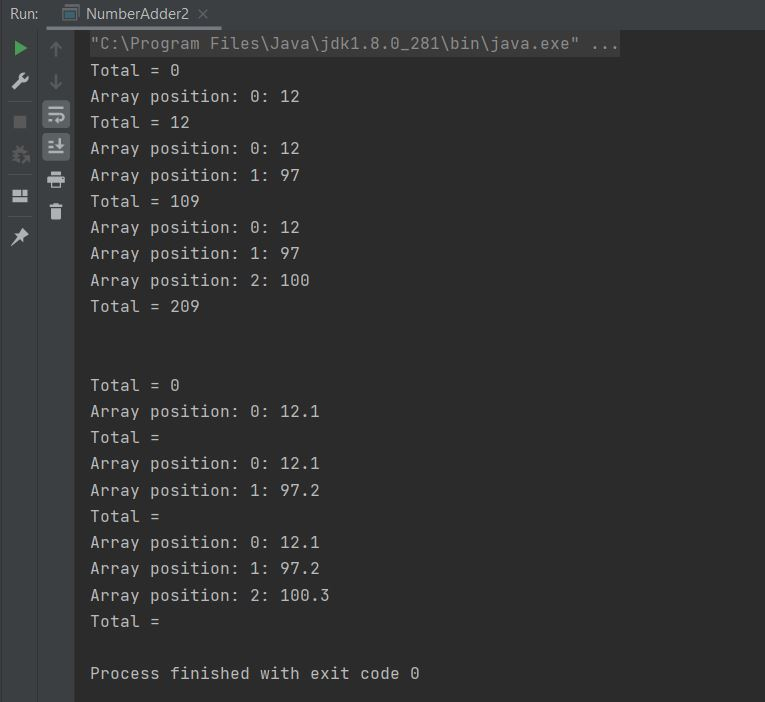The above screenshot shows the output of running our `NumberAdder2` class with overloaded methods. As you can see we can pass a variable number of arguments to the methods and the correct overloaded method is used.

#### Overloaded varargs Ambiguities

Now lets think about this, varargs seem the business and used correctly they are. But there are pitfalls you need to be aware of when overloading with varargs and we will give a couple of examples here. Lets write a new class to highlight an example:

``````
/*
Test class for maths stuff
*/
public class PrintStuff {

public static void main (String[] args) {
int a = 12;
printArray();  // Call with no arguments
printArray(a);  // Call with 1 arguments
String b = "fred" ;
printArray();  // Call with no arguments
printArray(b);  // Call with 1 arguments
}

/*
A method that Prints an array of ints
*/
static void printArray(int ... number) {
for (int i=0; i<number.length; i++) {
System.out.print("Array position: " + i + ": " + number[i]);
}
}

/*
A method that Prints an array of Strings
*/
static void printArray(String ... string) {
for (int i=0; i<string.length; i++) {
System.out.print("Array position: " + i + ": " + string[i]);
}
}
}

``````

Save and compile and the file in directory   c:\_ObjectsAndClasses in the usual way.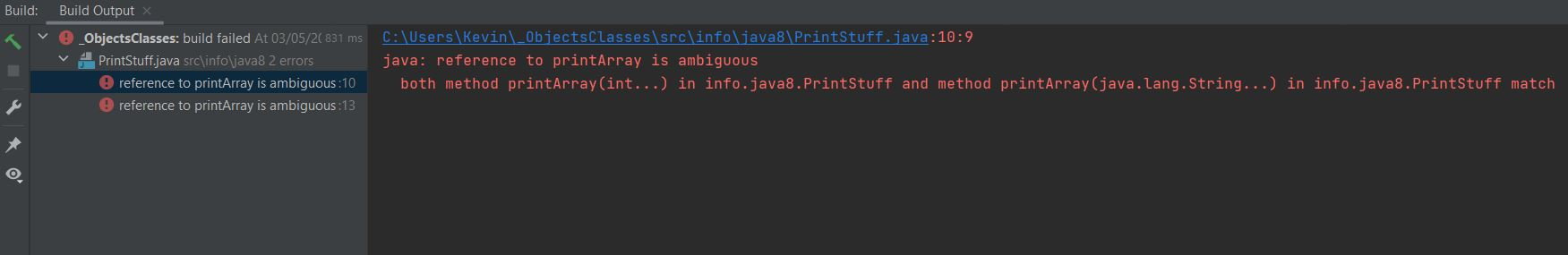The above screenshot shows the output of running our `PrintStuff` class with these overloaded methods. The compiler doesn't like this, but why?. Well lets think about it, when we pass the no-args list how can the compiler tell which method to go to, it can't so throws an ambiguous error.

Ok lets look at a differnt case with two parameters example:

``````
/*
Test class for maths stuff
*/
public class PrintStuff2 {

public static void main (String[] args) {
String a = "wilma" ;
printArray();  // Call with no arguments
printArray(a);  // Call with 1 arguments
String b = "fred" ;
printArray();  // Call with no arguments
printArray(b);  // Call with 1 arguments
}

/*
A method that Prints one or more String
*/
static void printArray(String ... string) {
for (int i=0; i<string.length; i++) {
System.out.print("Array position: " + i + ": " + string[i]);
}
}

/*
A method that Prints one or more String
*/
static void printArray(String singleString, String ... string) {
for (int i=0; i<string.length; i++) {
System.out.print("Array position: " + i + ": " + string[i]);
}
}
}

``````

Save and compile and the file in directory   c:\_ObjectsAndClasses in the usual way.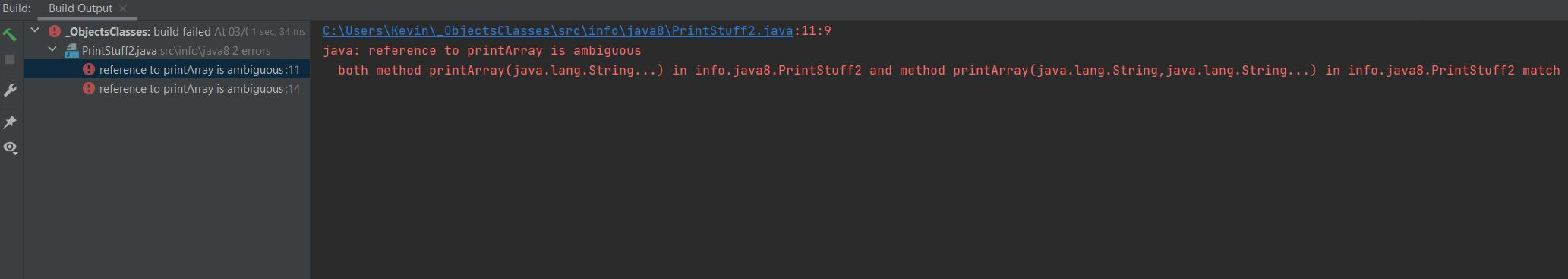The above screenshot shows the output of running our `PrintStuff2` class with these overloaded methods. The compiler doesn't like this, but why?. When we pass the 1-args list how can the compiler tell which method to go to, the single `String` method or the `String ... string ` method passing an empty array; it can't so throws an ambiguous error.

varargs are a great way to cut down our code, just be aware of the pitfalls when overloading them. Often when we get ambiguity like that shown above its a design flaw.

## Lesson 4 Complete

In this lesson we made a thorough investigation of methods and what we can do with these members.

### What's Next?

In the next lesson we look at instance variables and explain their scope.